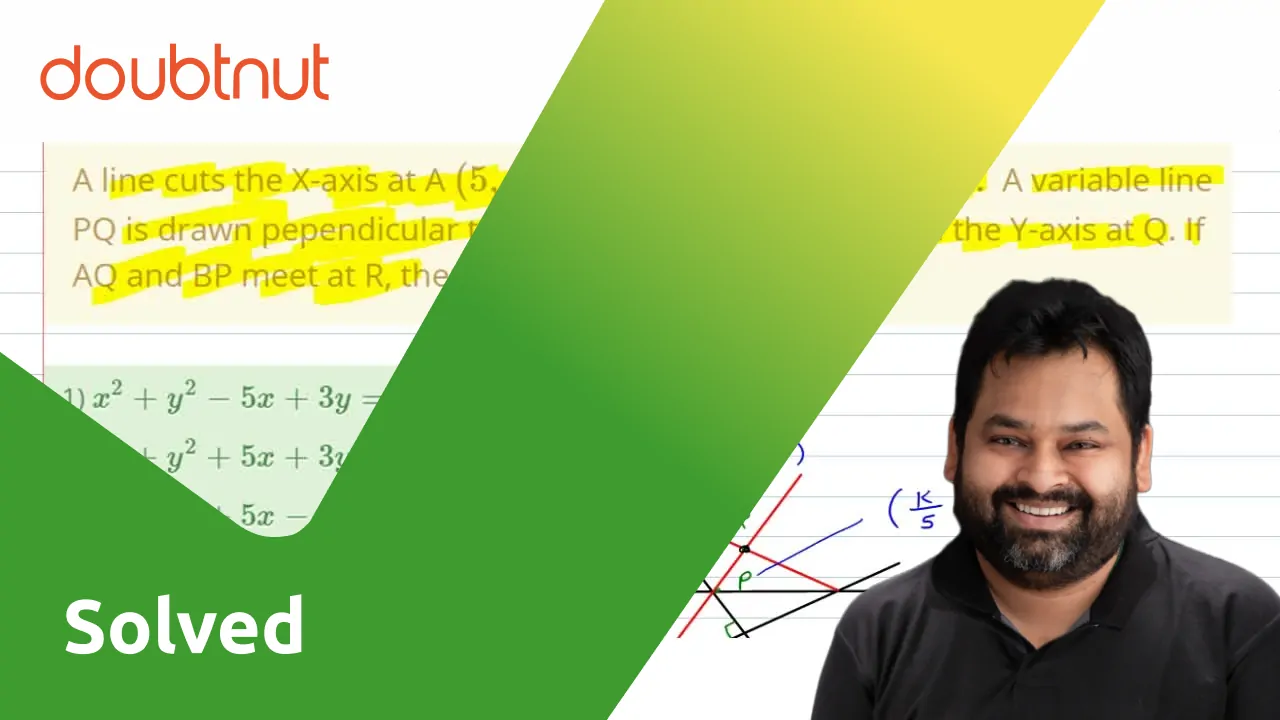Doubtnut App - Get 50 Lakh+ video solutions better than GoogleInstall Now

# A line cuts the X-axis at A (5,0) and the Y-axis at B(0,−3). A variable line PQ is drawn pependicular to AB cutting the X-axis at P and the Y-axis at Q. If AQ and BP meet at R, then the locus of R is Solution in Marathi

A

1) x2+y25x+3y=0

B

2) x2+y2+5x+3y=0

C

3) x2+y2+5x3y=0

D

4) x2+y25x3y=0

Text Solution
Verified by Experts

Video Solution